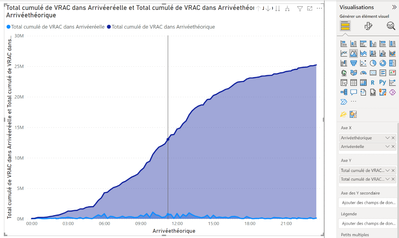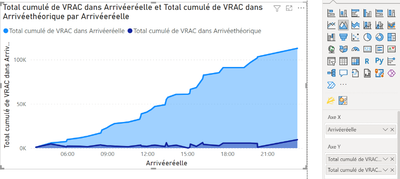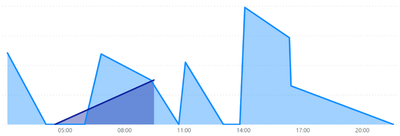cancel
Showing results for
Did you mean:Regular Visitor

## Create a area graph with two cumulative value with two x axis

Hi Power Community.

I am trying to create a area graph with two cumulative value on it, both based on a hour value.

Here is a sample of my date :

 theroerical time real time Quantity 14:00 14:37 10 15:00 15:05 5 16:00 15:10 25

I am using two measures to calculate my cumulative values, one for each hour :

``````CALCULATE(
SUM('QUANTITES Arrivée'[VRAC]),
FILTER(
ALLSELECTED('Quantity'[theroerical time]),
ISONORAFTER('Quantity'[theroerical time], MAX('Quantity'[theroerical time]), DESC)
)
)``````

For the other measure, replace reference to theroerical time to real time.

My issue is that i can't find a way to display my two measure in the same graph because it can't conciliate my two hours column on the same x axe.

I manage to go that far. One measure is correctly cumulated, the other one is not (because X axis doesn't correspond, despite being both a hour value)

My expected result would be to have both area cumulated.Is there a solution to allow both of my measure to be cumulated here ? My easiest take would be to concialiate the x axis but i failed to do it for the moment.

Thanks.

3 REPLIES 3Regular Visitor

I have created the TIMETABLE table and then made two relation :

• active relation Real Time with Time column from the new Timetable
• inactive relation Theroerical Time with Time column from the new Timetable

After that, I have added the USERELATIONSHIP in my cumulative sum measure (the other measure with active relation remain the same)

``````CALCULATE(
SUM('QUANTITES Arrivée'[VRAC]),
FILTER(
ALLSELECTED('QUANTITES Arrivée'[Arrivéethéorique]),
ISONORAFTER('QUANTITES Arrivée'[Arrivéethéorique], MAX('QUANTITES Arrivée'[Arrivéethéorique]), DESC)
),USERELATIONSHIP('QUANTITES Arrivée'[Arrivéethéorique],'TimeTable'[Time])
)``````

Despite this, the Theroerical Time still doesn't cumulate. Did I miss something ?Super User

@PBILP , for that you have to create a timetable. Join with both time columns and one join will be inactive, which you have to activate using userelationship

https://kohera.be/blog/power-bi/how-to-create-a-time-table-in-power-bi-in-a-few-simple-steps/

!! Microsoft Fabric !!
Microsoft Power BI Learning Resources, 2023 !!
Learn Power BI - Full Course with Dec-2022, with Window, Index, Offset, 100+ Topics !!
Did I answer your question? Mark my post as a solution! Appreciate your Kudos !! Proud to be a Super User! !!Regular Visitor

Hi again @amitchandak,

I tried again to use the document you provide me. I have include USERELATIONSHIP on both of my measures. Like this, as a first filter of my CALCULATE operation :

``````CALCULATE(
SUM('QUANTITES Arrivée'[VRAC]),
USERELATIONSHIP('QUANTITES Arrivée'[Arrivéethéorique],TimeTable[Time]),
FILTER(
ALLSELECTED('QUANTITES Arrivée'[Arrivéethéorique]),
ISONORAFTER('QUANTITES Arrivée'[Arrivéethéorique], MAX('QUANTITES Arrivée'[Arrivéethéorique]), DESC)
)
)``````

Then i use the TIMETABLE column Time in my area graph for the X axis.

This time, both of my value appears with the same axis. However, the value are not cumulated anymore as you can see in the picture below.

Do I need to adjust my measure or my graph do achieve to show my expected cumulated value on the same graph ?